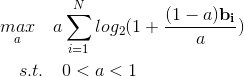# Semidefinite programming with rank 1 Hermitian semidefinite matric variable

#1

Dear experts, There is a convex SDP and it can be optimally solved by CVX. But due to the definitio`n of S=x*x' where 'x' is a complex column vector in communication field, t`he variable ‘S’ in the code below should be a Hermitian semidefinite matric with rank 1 . However, I have no idea to add the constraint of ‘rank(S)=1’. Here is the cvx code without the constraint of ‘rank(S)=1’:clear,clc;
g_k=0.01;
sigma_2=1.e-13;
n=4;
Q_max=0.1000;
P_max=1;
Beta_k=0.5;
Eta_k=0.5;
h_k=[0.0291 - 0.0047i, 0.0083 - 0.0027i, 0.0138 + 0.0110i, -0.0106 - 0.0028i];
cvx_begin sdp
variable Q_k
variable S(n,n) Hermitian semidefinite
maximize ( g_k.^2/sigma_2 * Q_k )
trace(S) <= P_max
Q_k<= Beta_k * Eta_k * h_k * S * h_k’ ;
Q_k<=Q_max; %#ok
cvx_end
S,Q_k,rank(S)

(Mark L. Stone) #2

`rank(S)=1` is a non-convex constraint, and can not be explicitly included in a problem presented to CVX.

AS you have seen, CVX may be able to handle a convex relaxation in which the rank constraint is relaxed to a convex constraint or eliminated altogether, or perhaps handled by minimizing a convex proxy, such as the nuclear norm.

#3

Thank you for your help, sir.

#5

Dear experts, I want to use -rel_entr(a,a+p) to solve the problem:where ‘a’ is a scalar variable, and ‘ b ’ is a known vector. I want to use -rel_entr(a,a+p) to solve the proplem above but I have no idea to solve the different sizes of ‘a’ and ‘p’. The wrong code is shown below.

``````b = 1.0e-14 * [0.0443    0.0267    0.1469];
cvx_begin
variable a
maximize -rel_entr(a,a*(1-b)+b)
0<a<1
cvx_end
``````

Thanks very much.

(Mark L. Stone) #6

Log2(x) = log(x)/log(2) so you can divide by log(2). However, the argmax would be the same even without dividing by log(2).

Change
`maximize -rel_entr(a,a*(1-b)+b)`
to
`maximize(sum(-rel_entr(a,a*(1-b)+b)`))
or if you really want log2, to
`maximize(sum(-rel_entr(a,a*(1-b)+b)`)/log(2))

The scaling of your problem may present difficulties. I’ll let you look into that.

#7

Thank you for your helpful response, sir. But I found that CVX usually mak mistake when the value of vector b is small enough. I plot the curve of the objective function versus variable ‘a’, and it can be easily seen that ‘a’ is around 0.01 when the maximal ‘obj’ is achieved. But CVX got a different optimal value of ‘a’(0.1690) which is obviously wrong. The result and code is shown below.

clear,close all
% the curve of ‘obj’ versus ‘a’;
%‘obj’------the objective function
%‘a’--------variable
b= 1.0e-03 [0.0573 0.1816 0.0567];
a=0:0.001:1;
for i=1:length(a)
R1=0;
for ii=1:3
R1=R1+log2(1+b(ii)
(1-a(i))/a(i));
end
obj(i)=a(i)*R1;
end
plot(a,obj)
xlabel({‘variable a’})
ylabel({‘the objective functione’})

cvx_begin
variable a
maximize(sum(-rel_entr(a,a*(1-b)+b)))
0<a<1
cvx_end
a

(Mark L. Stone) #8

That’is why I wrote
`The scaling of your problem may present difficulties. I’ll let you look into that.`

You need to improve the scaling of your problem. The solver apparently is not able to deal well with such small values of `b`.

Also, I rrecommend you install CVXQUAD per CVXQUAD: How to use CVXQUAD's Pade Approximant instead of CVX's unreliable Successive Approximation for GP mode, log, exp, entr, rel_entr, kl_div, log_det, det_rootn, exponential cone. CVXQUAD's Quantum (Matrix) Entropy & Matrix Log related functions . However, given that CVX’s Successive Approximation method appears to have succeeded, i doubt CVXQUAD will make your results come out better in this case, but I don’t know for sure.

Let’s see whether someone clever comes along who can come up with a nice change of variables or something to improve the formulation of the problem.so that the solver is presented a numeriically nicer problem.

#9

I will try your way and carefully check the scalling of the problem.Thanks very much for your help.